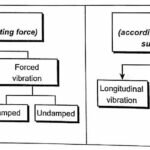# A Note on Simple Harmonic Motion – theteche.com

Since most of the vibrating system follow harmonic motion (SHM), therefore it is essential to have proper understanding of SHM related basic concepts.

A body is said to have simple harmonic motion (SHM), if it moves or vibrates about a mean position such that its acceleration is always proportional to its distance from the mean position and is directed towards the mean position or equilibrium position.

#### Differential Equation of SHM

Consider a particle ‘P’ moving around a circle with a uniform angular velocity ω rad/s as show in fig.Displacement of particle ‘P’ from mean position after time ‘t’, as shown in fig. is given by

$v=\frac{d x}{d t}=\omega \mathrm{X} \cos \omega t$

Acceleration of particle after time ‘t’ is

$\begin{array}{rlr} a=\frac{d^{2} x}{d t^{2}}=-\omega^{2} X \sin \omega t=-\omega^{2} x & \quad[\because x=X \sin \omega t] \\ \qquad \frac{d^{2} x}{d t^{2}}+\omega^{2} x=0 & \end{array}$

The above equation is known as differential equation or fundamental equation of S.H.M.

Time period and frequency:

Time Period, $t_{p}=\frac{2 \pi}{\omega}$

Frequency, $f=\frac{1}{t_{p}}=\frac{\omega}{2 \pi}$